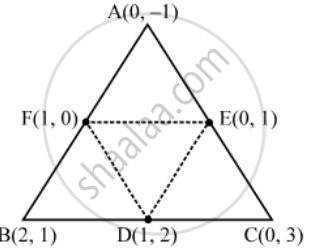# Find the Area of the Triangle Formed by Joining the Mid-point of the Sides of the Triangle Whose Vertices Are (0, –1), (2, 1) and (0, 3) - Mathematics

Sum

Find the area of the triangle formed by joining the mid-point of the sides of the triangle whose vertices are (0, –1), (2, 1) and (0, 3). Find the ratio of area of the triangle formed to the area of the given triangle.

#### Solution

Let A (0, –1), B(2, 1) and C(0, 3) be the vertices of ∆ABC.

Let D, E, F be the mid-points of sides BC, CA and AB respectively. Then, the coordinates of D, E and F are (1, 2), (0, 1) and (1, 0) respectively.

Now,

⇒ D=((0+2)/2,(-1+1)/2)=(1,0)

⇒ E =((0+0)/2+(3-1)/2)=(0,1)

⇒ F = ((2+0)/2,(1+3)/2)=(1,2)Area of triangle=1/2(x_1(y_2-y_3)+x_2(y_3-y_1)+x_3(y_1-y_2))

Area of Δ DEF =1/2(1(2-1)+1(1-0)+0(0-2))

=1 sq. units

Area of Δ ABC =1/2(0(1-3)+2{3-(-1)}+0(-1-1))

1/2{8}=4 sq. units

∴ Area of ∆DEF : Area of ∆ABC = 1 : 4

Concept: Area of a Triangle
Is there an error in this question or solution?